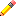#Boost C++ Libraries

...one of the most highly regarded and expertly designed C++ library projects in the world.

This is the documentation for an old version of boost. Click here for the latest Boost documentation.

Template classes can easily be exported too, but you can't export the template itself... you have to export instantiations of it! So, if you want to export a std::vector, you will have to export vectors of int, doubles, etc.

Suppose we have this code:

``` template <class T> struct Point { T x; T y; }; ```

And we want to export Points of int and double:

``` Point = Template("Point", "point.h") Point("int") Point("double") ```

Pyste will assign default names for each instantiation. In this example, those would be "Point_int" and "Point_double", but most of the time users will want to rename the instantiations:

``` Point("int", "IPoint") // renames the instantiation double_inst = Point("double") // another way to do the same rename(double_inst, "DPoint") ```

Note that you can rename, exclude, set policies, etc, in the Template object like you would do with a Function or a Class. This changes affect all future instantiations:

``` Point = Template("Point", "point.h") Point("float", "FPoint") // will have x and y as data members rename(Point.x, "X") rename(Point.y, "Y") Point("int", "IPoint") // will have X and Y as data members Point("double", "DPoint") // also will have X and Y as data member ```

If you want to change a option of a particular instantiation, you can do so:

``` Point = Template("Point", "point.h") Point("int", "IPoint") d_inst = Point("double", "DPoint") rename(d_inst.x, "X") // only DPoint is affect by this renames, rename(d_inst.y, "Y") // IPoint stays intact ```What if my template accepts more than one type? When you want to instantiate a template with more than one type, you can pass either a string with the types separated by whitespace, or a list of strings ("int double" or ["int", "double"] would both work).